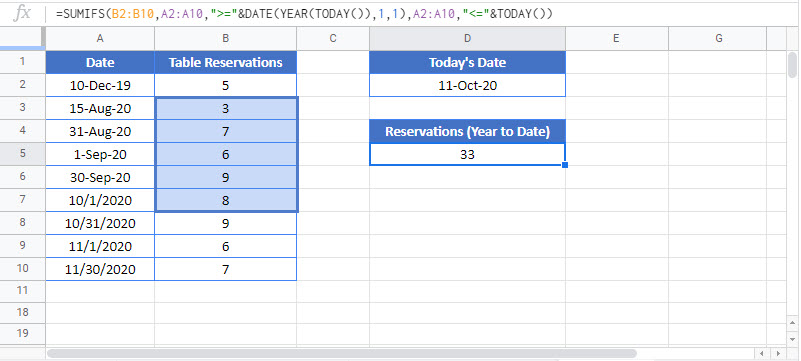# Sum By Month to Date or Year to Date- Excel & Google Sheets

This tutorial will demonstrate how to use the SUMIFS function to sum data month-to-date or year-to-date in Excel and Google Sheets.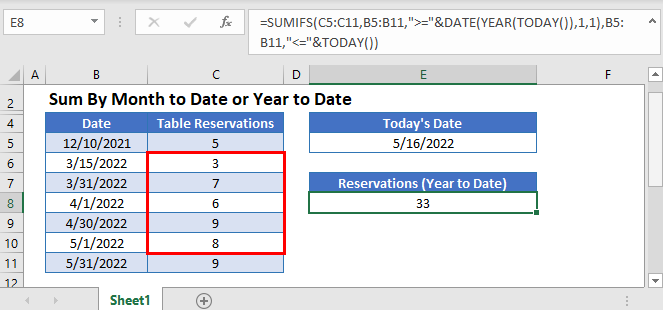## Sum by Month to Date

The SUMIFS Function sums data for rows that meet certain criteria. Its syntax is: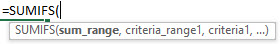This example sums Table Reservations month-to-date, using the Functions: SUMIFS, DATE, YEAR, MONTH, and TODAY.

``=SUMIFS(C3:C11,B3:B11,">="&DATE(YEAR(TODAY()),MONTH(TODAY()),1),B3:B11,"<="&TODAY())``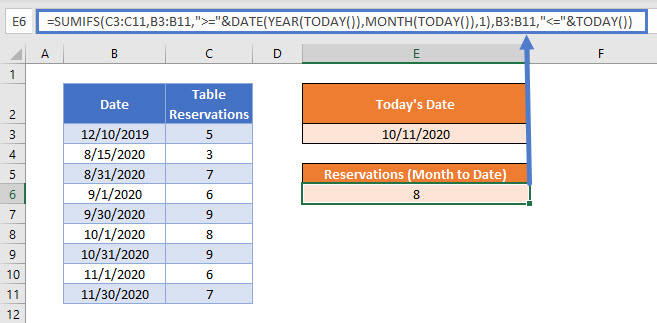Now let’s step through the formula.

### TODAY Function

The TODAY Function returns the current date. The value is recalculated each time the file is viewed.

``=TODAY()``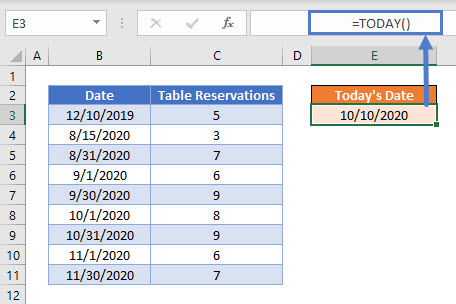This is the upper end of our date range for the formula.

### DATE Function

Then calculate the lower end of the date range: the first day of the current month. We use the DATE Function with YEAR and MONTH to produce this date value. Use the year and month of today’s date and set day to 1.

``=DATE(YEAR(E3),MONTH(E3),1)``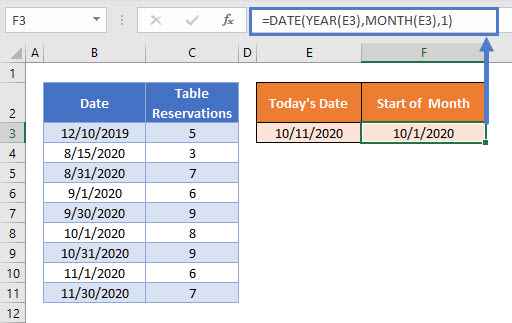### SUMIFS Function

Use the SUMIFS Function to sum Table Reservations when the date is greater than or equal to the first day of the month and less than or equal to the current day.

``=SUMIFS(C3:C11,B3:B11,">="&F3,B3:B11,"<="&E3)``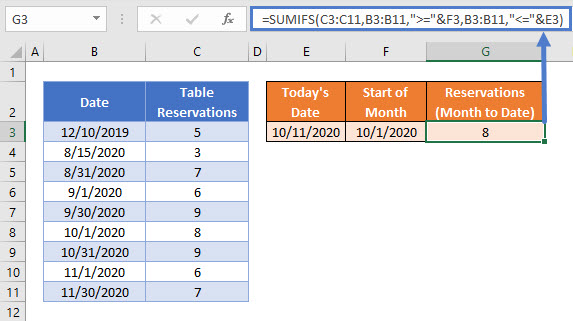Combining these steps gives us the formula for the month to date sum:

``=SUMIFS(C3:C11,B3:B11,">="&DATE(YEAR(TODAY()),MONTH(TODAY()),1),B3:B11,"<="&TODAY())``## Sum by Year to Date

The same logic can be used to sum the number of Table Reservations for the current year up until today by making the start date the first day of the year. Both month and day are set to 1.

``=SUMIFS(C3:C11,B3:B11,">="&DATE(YEAR(TODAY()),1,1),B3:B11,"<="&TODAY())``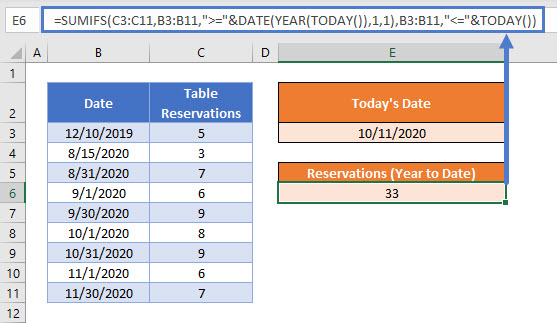## Locking Cell References

To make our formulas easier to read, we’ve shown them without locked cell references:

``=SUMIFS(C3:C11,B3:B11,">="&DATE(YEAR(TODAY()),1,1),B3:B11,"<="&TODAY())``

But these formulas will not work properly when copy and pasted elsewhere with your Excel file. Instead, you should use locked cell references like this:

``=SUMIFS(\$C\$3:\$C\$11,\$B\$3:\$B\$11,">="&DATE(YEAR(TODAY()),1,1),\$B\$3:\$B\$11,"<="&TODAY())``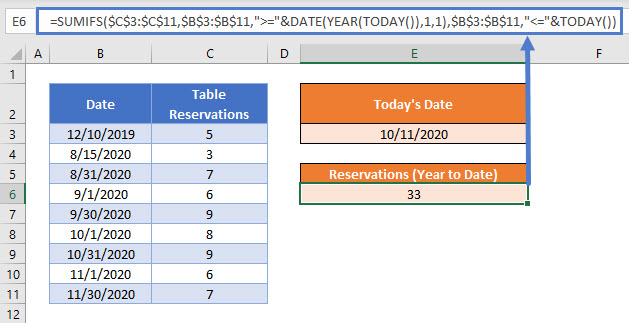### Easy Excel Automation